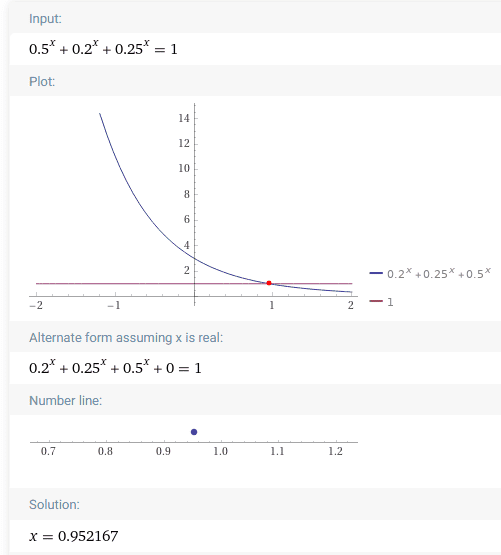# Is there a formula to get x in Excel without using solver: 0.5^x+0.2^x+0.25^x=1

The Investor
TL;DR Summary
Hi! I wanted to see if there is a formula to get x in excel without using solver: 0.5^x+0.2^x+0.25^x=1. Thanks!
0.5^x+0.2^x+0.25^x=1.

Homework Helper
Gold Member
I don't think there is a neat formula for x.
https://www.wolframalpha.com/input/?i=0.5^x+0.2^x+0.25^x=1•morrobay and berkeman
Mentor
There is no analytic solution to it and it's not a standard problem, so you need some sort of iterative approach. You can do that with the solver, you can write a macro to do it, you can even do it in some Excel cells (e.g. using the Newton method), but Excel won't have a nice formula for it.

•berkeman
The Investor
OK, that's what I thought. Cheers guys.